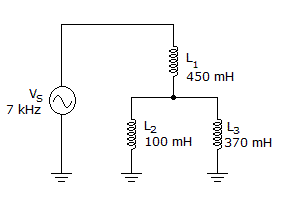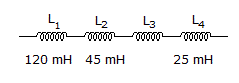# Electronics - Inductors

### Exercise :: Inductors - Filling the Blanks

6.The total inductive reactance is ___ in the given circuit.

 A. 3.5 kB. 4.4 kC. 16.3 kD. 23.3 kExplanation:

No answer description available for this question. Let us discuss.

7.The inductance value of L3 in the given figure is _____ if the total inductance is 340 mH.

 A. 150 mH B. 220 mH C. 295 mH D. 315 mH

Explanation:

No answer description available for this question. Let us discuss.

8.

When current flow tries to decrease in an inductor, the magnetic field _______________ and the voltage polarity across the inductor _______________.

 A. collapses, remains the same B. expands, reverses C. collapses, reverses D. expands, remains the same

Explanation:

No answer description available for this question. Let us discuss.

9.

Electromagnetic induction is the generation of _________ from __________.

 A. magnetism, electricity B. electricity, electricity C. electricity, magnetism D. magnetism, magnetism

Explanation:

No answer description available for this question. Let us discuss.

10.

Inductive ___ represents the opposition to an alternating current.

 A. impedance B. resistance C. reluctance D. reactance